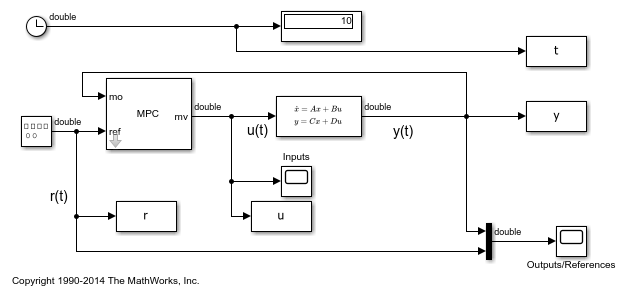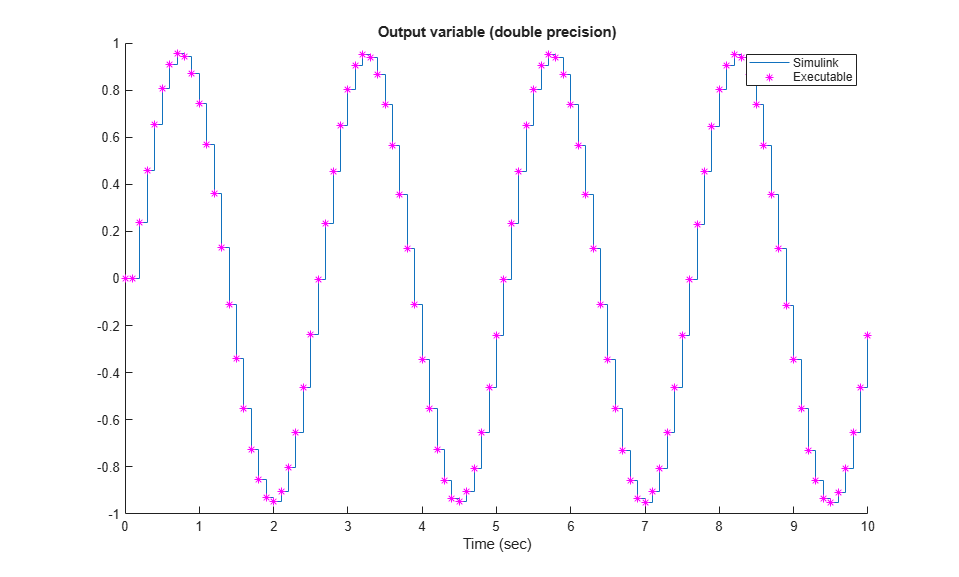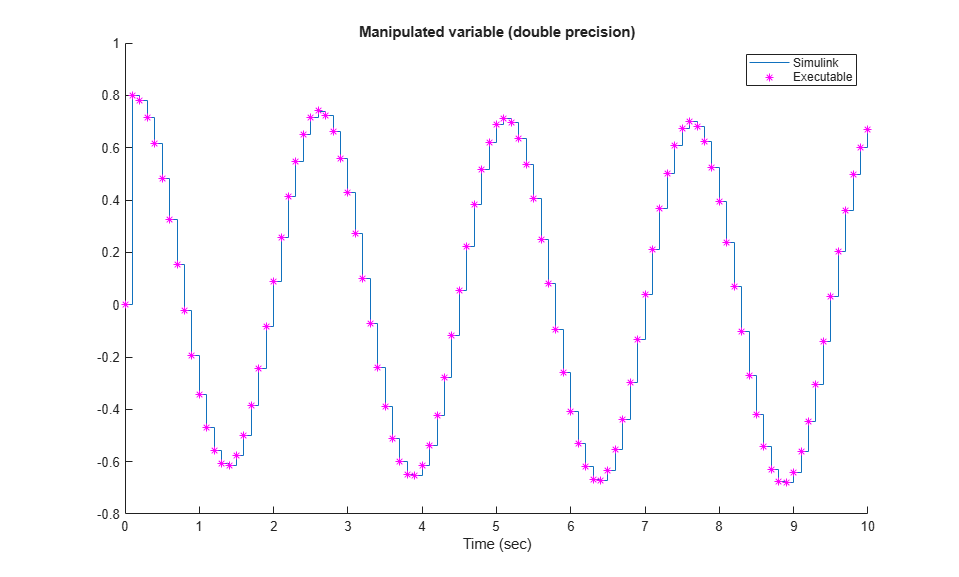# Simulation and Code Generation Using Simulink Coder

This example shows how to simulate and generate real-time code for an MPC Controller block with Simulink® Coder™. Code can be generated in both single and double precisions.

### Define Plant Model and MPC Controller

Define a SISO plant.

```plant = ss(tf([3 1],[1 0.6 1])); ```

Define the MPC controller for the plant.

```Ts = 0.1; %Sample time p = 10; %Prediction horizon m = 2; %Control horizon % Define weights along with input and output constraints. Weights = struct(MV=0, MVRate=0.01, OV=1); MV = struct(Min=-Inf, Max=Inf, RateMin=-100, RateMax=100); OV = struct(Min=-2, Max=2); % Define mpc object mpcobj = mpc(plant,Ts,p,m,Weights,MV,OV); ```

### Simulate and Generate Code in Double-Precision

By default, MPC Controller blocks use double-precision data for simulation and code generation.

```mdl1 = "mpc_rtwdemo"; open_system(mdl1) sim(mdl1) ```
```-->Converting model to discrete time. -->Assuming output disturbance added to measured output #1 is integrated white noise. -->"Model.Noise" is empty. Assuming white noise on each measured output. ```The controller effort and the plant output are saved into base workspace as variables `u` and `y`, respectively.

Build the model with the `slbuild` command. Use `evalc` to capture the text output for possible later inspection.

```set_param(mdl1,RTWVerbose="off") disp("Generating C code... Please wait until done.") txt_out_double = evalc("slbuild(mdl1);"); ```
```Generating C code... Please wait until done. ```

On a Windows® system, an executable file named `mpc_rtwdemo.exe` appears in the current folder after the build process finishes.

Run the executable.

```status = system("." + filesep + mdl1); ```
```** starting the model ** ** created mpc_rtwdemo.mat ** ```

After the executable completes successfully (status=0), a data file named `mpc_rtwdemo.mat` appears in the current directory. This file contains the results from running the executable file. Load the mat file.

```load(mdl1) ```

Compare the responses from the executable (`rt_u` and `rt_y`) with the responses from the previous simulation in Simulink (`u` and `y`).

```% Manipulated variable figure hold on stairs(t,u) stairs(rt_t,rt_u,"*m") xlabel("Time (sec)") title("Manipulated variable (double precision)") legend("Simulink","Executable") hold off % Output variable figure hold on stairs(t,y) stairs(rt_t,rt_y,"*m") xlabel("Time (sec)") title("Output variable (double precision)") legend("Simulink","Executable") hold off ```Display the norm of the differences.

```% Manipulated variable norm(u-rt_u) % Output Variable norm(y-rt_y) ```
```ans = 1.1992e-13 ans = 2.3693e-14 ```

The responses are numerically equal.

### Simulate and Generate Code in Single-Precision

You can also configure the MPC block to use single-precision data in simulation and code generation.

```mdl2 = "mpc_rtwdemo_single"; open_system(mdl2) ```To do so, set the Output data type property of the MPC Controller block to `single`.

```sim(mdl2) ```

The controller effort and the plant output are saved into base workspace as variables `u1` and `y1`, respectively.

Build the model with the `slbuild` command. Use `evalc` to capture the text output for possible later inspection.

```set_param(mdl2,RTWVerbose="off") disp("Generating C code... Please wait until done.") txt_out_single = evalc("slbuild(mdl2);"); ```
```Generating C code... Please wait until done. ```

On a Windows system, an executable file named `mpc_rtwdemo_single.exe` appears in the temporary directory after the build process finishes

Run the executable.

```status = system("." + filesep + mdl2); ```
```** starting the model ** ** created mpc_rtwdemo_single.mat ** ```

After the executable completes successfully (status=0), a data file named `mpc_rtwdemo_single.mat` appears in the temporary directory. Load the mat file.

```load(mdl2) ```

Compare the responses from the generated code (`rt_u1` and `rt_y1`) with the responses from the previous simulation in Simulink (`u1` and `y1`).

```% Manipulated variable figure hold on stairs(t,u1) stairs(rt_t,rt_u1,"*m") xlabel("Time (sec)") title("Manipulated variable (double precision)") legend("Simulink","Executable") hold off % Output variable figure hold on stairs(t,y1) stairs(rt_t,rt_y1,"*m") xlabel("Time (sec)") title("Output variable (double precision)") legend("Simulink","Executable") hold off ```Display the norm of the differences.

```% Manipulated variable norm(u1-rt_u1) % Output Variable norm(y1-rt_y1) ```
```ans = single 5.2409e-05 ans = 1.0996e-05 ```

The responses are still practically equal, despite the fact that using a single numeric representation results in the norm of the response difference that is several order of magnitude larger.